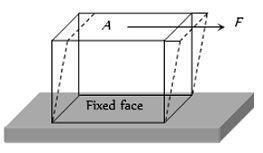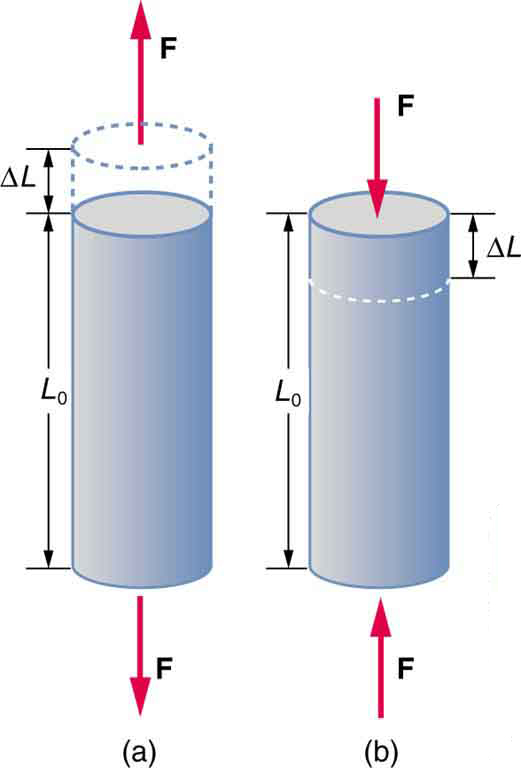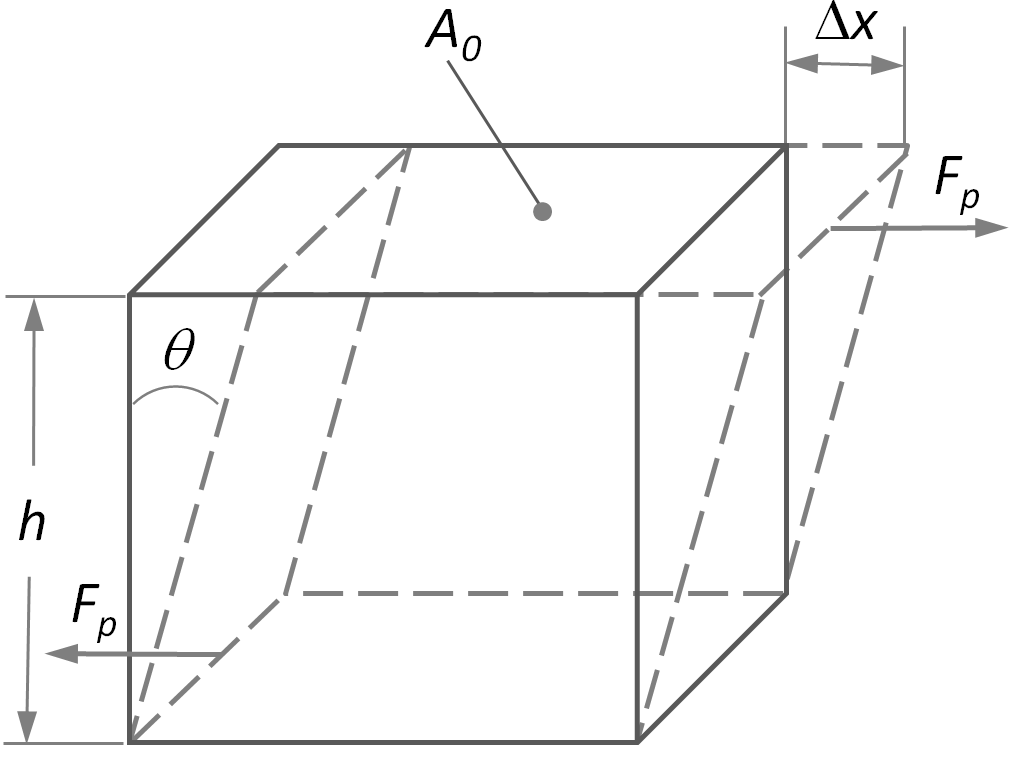Introduction of Elasticity﻿↪ Elasticity is a fundamental property of all matter by virtue of which an object regains its original shape and size when a deforming force is removed.↪ When the deforming force is applied to this object, it displaces the molecules of the object from their original states.

↪ Consequently, a force of reaction called restoring force is produced.

↪ This is due to the intermolecular forces with which the object tends to regain its original state.

↪ By the deforming force we mean that it is an external force being applied to an object to make it deform.

⁕ ﻿Difference between Elasticity and PlasticityElasticity Plasticity 1. Elasticity is the property of an object by virtue of which it can regain its original shape and size when the deforming force is removed. 2. Plasticity is the property of an object by virtue of which it can't regain its original shape and size when the deforming force is removed. 2. For the same amount of a deforming force, less extension is produced in it. 2. For the same amount of a deforming force, more extension is produced in it. 3. Elastic object follows Hooke's law within elastic limit. 3. Plastic object doesn't follow Hooke's law.

⁕ Stress↪ ﻿The internal restoring or elastic force acting per unit area of a deformed object is called stress.

↪ Since in equilibrium, the internal restoring force is equal in magnitude to the deforming force, the stress is measured as the deforming force per unit area of the object.

That is,⁕ ﻿Types of Stress

﻿

• ﻿Normal Stress

↪ If the deforming force is applied normally to the surface of an object, the stress is called normal stress.

↪ The stretching of a rod or a wire by pulling its ends produces the tension in it.

↪ So the normal stress is also called tensile stress.

• Tangential Stress↪ If the deforming force is tangential to the surface of an object, the stress is called tangential stress.

↪ This is seen when an object is twisted.

• Bulk Stress or volume stress

↪ Bulk stress is defined as the force acting perpendicular to the surface of the object per unit area.﻿⁕ Strain↪ ﻿The change in size or shape of an object due to the deforming force is called strain and is measured as the ratio of change in size of the object to the original size.

• Longitudinal Strain

↪ It is defined as the change in length per unit original length. It is also known as tensile strain. That is,• Volume Strain↪ It is defined as change in volume per unit original volume. That is,• Shear Strain↪ It is defined as the angle θ through which a line originally perpendicular to a fixed face is turned. The angle is called the angle of shear.